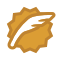# Number Sequence

Time Limit: 2000/1000 MS (Java/Others)    Memory Limit: 65536/32768 K (Java/Others)
Total Submission(s): 89480    Accepted Submission(s): 21333

Problem Description
A number sequence is defined as follows:

f(1) = 1, f(2) = 1, f(n) = (A * f(n - 1) + B * f(n - 2)) mod 7.

Given A, B, and n, you are to calculate the value of f(n).

Input
The input consists of multiple test cases. Each test case contains 3 integers A, B and n on a single line (1 <= A, B <= 1000, 1 <= n <= 100,000,000). Three zeros signal the end of input and this test case is not to be processed.

Output
For each test case, print the value of f(n) on a single line.

Sample Input
1 1 3 1 2 10 0 0 0

Sample Output
2 5

#include<iostream>
using namespace std;

int f(int a, int b, int n)
{
if(n == 1 )
return 1;
else if(n == 2)
return 1;
else return (a*f(a , b,n - 1) + b*f(a, b,n - 2))%7 ;
}
int main()
{
int a, b, n;
while(cin>>a>>b>>n)
{
if(a == 0 && b == 0 && n ==0)
{
return 0;
}
cout<<f(a, b, n)<<endl;
}
return 0;
}


wangxiaomingCSDN认证博客专家 架构 Spring Boot Redis

12-23107

#### HDU1005-数字序列09-03284

#### HDU1005（矩阵快速幂）

02-13691

#### hdu acm1005

09-195056

#### HDU-1005

10-18429

#### hdu 1005 简单题

02-151371

#### 【HDU1005】Number Sequence(矩阵快速幂)©️2020 CSDN 皮肤主题: Age of Ai 设计师: meimeiellie点击重新获取扫码支付1.余额是钱包充值的虚拟货币，按照1:1的比例进行支付金额的抵扣。
2.余额无法直接购买下载，可以购买VIP、C币套餐、付费专栏及课程。余额充值